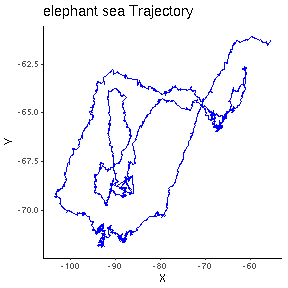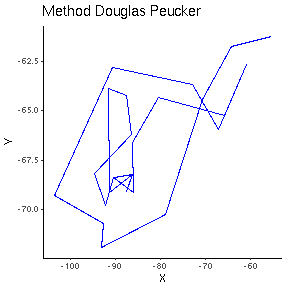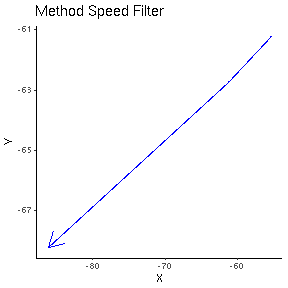TrajDataMining

The TrajDataMining contains a set of methods for trajectory data preparation, such as filtering, compressing and clustering, and for trajectory pattern discovery. The meth- ods for data preparation are important to prepare trajectory data sets before the data mining phase. TrajDataMining contains the following methods:

• A speed filter that filters out trajectory observations whose speeds are above a user-defined maximum velocity ( ETIENNE , 2011).

• Two compression algorithms: (1) Douglas-Peucker which reduces trajectories by preserving spatial precisions ( DOUGLAS; PEUCKER , 1973), and (2) Open-window Meratnia-By which reduces trajectories by preserving spatiotempo- ral precisions ( MERATNIA; BY , 2004).

• Two algorithms to discover when objects stop and move, CB-SMoT ( PALMA et al. , 2008) and DB-SMoT ( ROCHA et al. , 2010), which can be used to semanti- cally enrich the trajectory data.

• A method, named Partner, that identifies objects that are moving together. We propose this method to recognize trajectories that stay together, based on trajectory distance time series analysis.

Example data gives

library(TrajDataMining)
library(ggplot2)
library(sp)
library(magrittr)
suppressMessages(library(rgdal))

Plot of A1 data example

A1 track its a trajectory of sea elephant

df <- data.frame(x=A1@sp@coords[,1],y=A1@sp@coords[,2])

ggplot(df,aes(x=df\$x,y=df\$y))+geom_path(aes(group = 1), arrow = arrow(),color='blue')+ggtitle("elephant sea Trajectory")+xlab("X")+ ylab("Y")+theme_classic()Douglas Peucker Method

Method that reduces a trajectory spatially

# get the maximum distance
max <- max(A1@connections\$distance)

douglasp <- douglasPeucker(A1,max)

df <- data.frame(x=douglasp@sp@coords[,1],y=douglasp@sp@coords[,2])
ggplot(df,aes(x=df\$x,y=df\$y))+geom_path(aes(group = 1), arrow = arrow(),color='blue')+ggtitle("Method Douglas Peucker")+xlab("X")+ ylab("Y")+theme_classic()Speed Filter

Method removes regions where speed was higher than the defined parameter

# get the maximum distance
sf <- speedFilter(A1,0.01)
##  "2008-01-23 21:32:44"
##  "2008-01-24 01:31:26"
##  "2008-01-24 03:12:00"
##  "2008-01-24 11:24:42"
##  "2008-07-21 04:30:30"
##  "2008-07-21 11:22:30"
##  "2008-07-22 01:09:49"
##  "2008-07-25 13:19:46"
##  "2008-07-28 11:40:23"
##  "2008-07-29 01:23:43"
##  "2008-07-31 09:15:41"
##  "2008-11-19 12:38:34"
df <- data.frame(x=sf@sp@coords[,1],y=sf@sp@coords[,2])

ggplot(df,aes(x=df\$x,y=df\$y))+geom_path(aes(group = 1), arrow = arrow(),color='blue')+ggtitle("Method Speed Filter")+xlab("X")+ ylab("Y")+theme_classic()Partner

Method to identify if two trajectories are partners

For to send this data for the database use the class DataSourceInfo.

data <- FALSE

partner(A1,A2,110792,2277,0,data)
##             begintime             endtime id1 id2
## 1 2008-02-04 21:26:54 2008-11-11 06:58:44   1   2